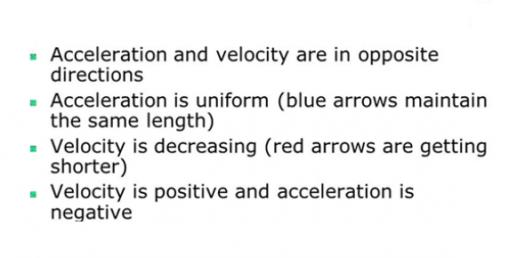# Velocity And Acceleration Test! Physics Trivia Questions Quiz

5 Questions | Total Attempts: 22Settings.

Related Topics
• 1.
While a car travels around a circular track at a constant speed, its
• A.

Acceleration is zero

• B.

Velocity is zero

• C.

Both a and b

• D.

None of the above

• 2.
Acceleration is defined as
• A.

Rate of displacement.

• B.

The rate of change of displacement.

• C.

The change in velocity.

• D.

The rate of change of velocity.

• 3.
If you know a car’s acceleration, the information you must have to determine if the car’s velocity is increasing is the
• A.

Direction of the car’s initial velocity.

• B.

Direction of the car’s acceleration.

• C.

Initial speed of the car.

• D.

Final velocity of the car.

• 4.
If you know the acceleration of a car and its initial velocity, you can predict which of the following?
• A.

The direction of the car’s final velocity

• B.

The magnitude of the car’s final velocity

• C.

The displacement of the car

• D.

All of the above

• 5.
When a car’s velocity is positive and its acceleration is negative, what is happening to the car’s motion?
• A.

The car slows down.

• B.

The car speeds up.

• C.

The car travels at constant speed.

• D.

The car remains at rest.